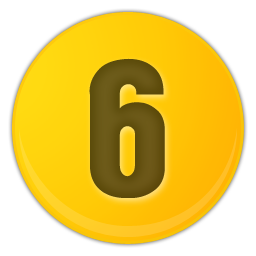Language: English | España | РусскийShare image:

Keywords: Number 6 PNG size: 256x256px, 6 PNG images, PNG image: Number 6 PNG, free PNG image, 6
Image category: 6
Format: PNG image with alpha (transparent)
Resolution: 256x256
Size: 41 kb

# Number 6 PNG image with transparent background | number6_PNG18605.png

This image has format transparent PNG with resolution 256x256.

# 6

6 (six) is the natural number following 5 and preceding 7. It is a composite number and the smallest perfect number.

6 is the smallest positive integer which is neither a square number nor a prime number. Six is the second smallest composite number; its proper divisors are 1, 2 and 3.

Since six equals the sum of its proper divisors, six is the smallest perfect number, Granville number, and {\displaystyle {\mathcal {S}}}{\mathcal {S}}-perfect number.

As a perfect number:

6 is related to the Mersenne prime 3, since 21(22 – 1) = 6. (The next perfect number is 28.)

6 is the only even perfect number that is not the sum of successive odd cubes.

6 is the root of the 6-aliquot tree, and is itself the aliquot sum of only one number; the square number, 25.

Six is the only number that is both the sum and the product if three consecutive positive numbers.

Unrelated to 6's being a perfect number, a Golomb ruler of length 6 is a "perfect ruler". Six is a congruent number.